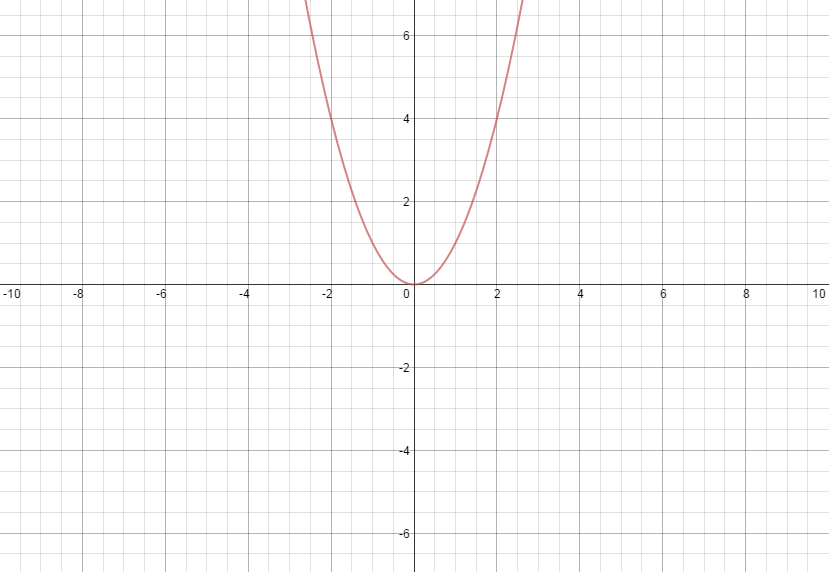## oliverpope

computer scientist

# What are Algorithms?

An algorithm is something every person does every single day, it is the procedure or formula for solving a problem. For example, when an average person wakes up they will take a shower, do their hair, eat breakfast, drink coffee, watch the news, get dressed, and go to work. You could specify this as a person’s algorithm for getting ready for their day, it is simply the procedure one goes through to solve one’s problem.

In mathematics, an algorithm can simply be a function, such as $f(x)= x^2$. Where the procedure is finding where $y=0$ and that would be $x$. Such as this equation would be 0, as shown below.In computer science, an algorithm can be a lot harder to understand or interpret, however it is mostly the same thing. If, for instance, I wanted to know if 5 was greater than 1, I could use JavaScript to do it:

// simple if statement
if (5 > 1) {
console.log("5 is greater than 1");
} else {
// if 5>1 was an inequality, you could handle it with an else statement, however this is optional
console.log("this will never happen");
}


Algorithms can get much more complicated and hardcore than these simple examples, applications and programs are just elaborate algorithms that use special things like an if statement to make magic happen. All it is is common sense and logic, literally everyone does it.